Next: 9.13 Programming Assignments Up: 9. Sorting Methods Previous: 9.11 To Probe Further

# 9.12 Problems

1.
Consider the following sorting methods: Bubble sort, Insertion Sort, Selection sort, Shell sort, Merge sort, Quick sort, and Heap sort.
• Sort the following keys using each of the above methods:
22, 36, 6, 79, 26, 45, 2, 13, 31, 62, 10, 79, 33, 11, 62, 26
• A sorting algorithm is said to be stable if it preserves the original order of records with equal keys. Which of the above methods are stable?
• Suppose you are to sort a list L comprising a sorted list followed by a few random elements. Which of the above sorting methods would you prefer and why?
2.
Show that if k is the smallest integer greater than or equal to n + log2n - 2, k comparisons are necessary and sufficient to find the largest and second largest elements of a set of n distinct elements.
3.
Design an algorithm to find the two smallest elements in an array of length n. Can this be done in fewer than 2n - 3 comparisons?
4.
Show how to find the minimum and maximum elements in an array using only (2n - 3) comparisons, where n is the size of the array.
5.
Show that any sorting algorithm that moves elements only one position at a time must have time complexity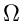(n2).
6.
Design an efficient algorithm to find all duplicates in a list of n elements.
7.
Find a sorting method for four keys that is optimal in the sense of doing the smallest possible number of key comparisons in the worst case. Find how many comparisons your algorithm does in the average case.
8.
Suppose we have a set of words, i.e., strings of the letters a-z, whose total length is n. Show how to sort these in O(n) time. Note that if the maximum length of a word is constant, then bin sort will work. However, you must consider the case where some of the words are very long.
9.
Suppose we have a sorted array of strings s1,..., sn. Write an algorithm to determine whether a given string x is a member of this sequence. What is the time complexity of your algorithm as a function of n and the length of x?
10.
Design an algorithm that will arrange a contiguous list of real numbers so that all the items with negative keys will come first, then those with nonnegative keys. The final list need not be sorted.
11.
Design an algorithm that will rearrange a list of integers as described in each case below.
• All even integers come before all odd integers.
• Either all the integers in even-numbered positions will be even or all integers in the odd-numbered positions will be odd. First, prove that one or the other of these goals can be achieved, although it may not be possible to achieve both goals at the same time.
12.
Suppose that the splits at every level of quicksort are in the proportion 1 -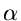to, where 0 <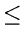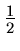andis a constant. Show that the minimum depth of a leaf in the recursion tree of quicksort is approximately -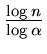and the maximum depth is approximately -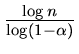. (Ignore the integer round off).
13.
Recall the algorithm Select(i, j, k) that finds the kth element in the sorted order of the elements a[i], a[i + 1],..., a[j]. Choose the pivot as follows. Divide the elements into groups of 3 (leaving aside between 0 and 2 elements that cannot be placed in a group), sort each group, and take the middle element from each group; a total of say, p, middle elements will result. Choose the median of these p elements as the pivot. Let T(n) be the time taken by a call to Select on n elements. Write down an appropriate recurrence relation for T(n). Is T(n), O(n)?
14.
In the above problem, choose the pivot as follows. Divide the elements into groups of 7, leaving aside between 0 and 6 elements that cannot be placed in a group. Sort each group and take the middle element from each group. Choose the median of these middle elements as the pivot.

Let T(n) be the time taken by a call to select on n elements. Write down an appropriate recurrence for T(n) and show that it is O(n).

15.
A d-ary heap is like a binary heap, but in stead of two children, nodes have d children.
• How would you represent a d-ary heap in an array?
• What is the height of a d-ary heap of n elements in terms of n and d?
• Give an efficient implementation of deletemin and insert operations and analyze the running time in terms of d and n.
16.
Consider constructing a heap by first forming a complete binary tree and then repeatedly applying the pushdown procedure. What input permutations of (1, 2, 3, 4, 5) are transformed into (1, 2, 4, 3, 5) by this process?
17.
Give a permutation of 1, 2,..., 8, which when input to the quicksort algorithm will produce the best possible performance of the quicksort algorithm (assume that the larger of the first two keys is selected as the pivot for partitioning).
18.
Suppose we have an array of n data records such that the key of each record has the value 0 or 1. Outline a worst case linear time algorithm to sort these records in place, using only an additional amount of storage equal to that of one record. Is your algorithm stable? Justify.
19.
Outline an O(nlog k) algorithm to merge k sorted lists into a single sorted list, where n is the total number of elements in all the input lists.
20.
Outline an efficient algorithm, using the binomial queue data structure to merge k sorted lists into a single sorted list. What is the worst-case complexity of your algorithm (in terms of k and n), if n is the total number of elements in all the input lists.
21.
Suppose we have an array of n data records such that the key of each record has the value 0, 1, or 2. Outline a worst case linear time algorithm to sort these records in place, using only an additional amount of storage equal to that of one record. Is your algorithm stable? Justify.
22.
Write down a decision tree for sorting three elements a, b, c using bubble sort, with proper annotations on the nodes and edges of the tree.Next: 9.13 Programming Assignments Up: 9. Sorting Methods Previous: 9.11 To Probe Further
eEL,CSA_Dept,IISc,Bangalore